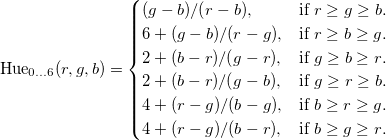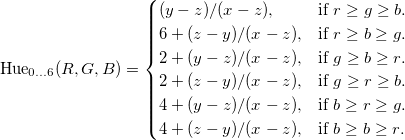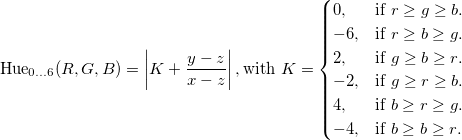Posts for the month of January 2013

# A fast RGB to HSV floating point conversion

The operations typically performed to convert from RGB to HSV are the following:

• find the largest RGB component
• find the smallest RGB component
• compute V and S
• select the main circular sector for H
• compute H

Here is, to my knowledge, the most commonly used RGB to HSV routine for floating point, with an extra minor optimisation (adding 1e-20f to divisors to avoid the need to care about divisions by zero):

static void RGB2HSV(float r, float g, float b,
float &h, float &s, float &v)
{
float rgb_max = std::max(r, std::max(g, b));
float rgb_min = std::min(r, std::min(g, b));
float delta = rgb_max - rgb_min;
s = delta / (rgb_max + 1e-20f);
v = rgb_max;

float hue;
if (r == rgb_max)
hue = (g - b) / (delta + 1e-20f);
else if (g == rgb_max)
hue = 2 + (b - r) / (delta + 1e-20f);
else
hue = 4 + (r - g) / (delta + 1e-20f);
if (hue < 0)
hue += 6.f;
h = hue * (1.f / 6.f);
}


Several things seem worth noticing already:

• Most of the complexity comes from the hue calculation.
• Four min/max operations are performed to find rgb_max and rgb_min; however, sorting three values can be done with only 3 comparisons. This is not necessarily problematic because min/max could be wired in an efficient way depending on the CPU.
• Two additional tests are performed to compare r and g to rgb_max; if rgb_max and rgb_min were computed using tests, this is a waste of time to compare them again.
• Adding 6.f to the final hue value only has a 16.6% chance of happening.

The actual hue calculation depends on how r, g, and b are ordered:But let’s rewrite this in terms of x, y and z, where x is the largest of (r,g,b), z is the smallest of the three, and y is inbetween:There are a lot of similarities here. We can push it even further, using the fact that x ≥ z and y ≥ z by definition:That’s actually the same calculation! Only the hue offset K changes. The idea now is the following:

• Sort the triplet (r,g,b) using comparisons
• Build K while sorting the triplet
• Perform the final calculation

Putting the idea into practice gives us the following code:

static void RGB2HSV(float r, float g, float b,
float &h, float &s, float &v)
{
float K = 0.f;

if (g < b)
{
float tmp = g; g = b; b = tmp;
K = -1.f;
}

if (r < g)
{
float tmp = r; r = g; g = tmp;
K = -2.f / 6.f - K;
}

if (g < b)
{
float tmp = g; g = b; b = tmp;
K = -K;
}

float chroma = r - b;
h = fabs(K + (g - b) / (6.f * chroma + 1e-20f));
s = chroma / (r + 1e-20f);
v = r;
}


You can check for yourself that the values for K explicited above are properly generated by that function. There were many other ways to sort (r,g,b) but this specific one lets us do one final optimisation.

We notice that the last swap effectively changes the sign of K and the sign of g - b. Since both are then added and passed to fabs(), the sign reversal can actually be omitted.

That additional trickery gives us this final code:

static void RGB2HSV(float r, float g, float b,
float &h, float &s, float &v)
{
float K = 0.f;

if (g < b)
{
std::swap(g, b);
K = -1.f;
}

if (r < g)
{
std::swap(r, g);
K = -2.f / 6.f - K;
}

float chroma = r - std::min(g, b);
h = fabs(K + (g - b) / (6.f * chroma + 1e-20f));
s = chroma / (r + 1e-20f);
v = r;
}


That’s 2 tests and 1 std::min call instead of the previous 3 tests and 4 std::min/max calls. We really should see some kind of performance gain here.

And as expected, benchmarks indicate a performance increase of 25 to 40 % with a great variety of CPUs, compilers and compiler flags. The following graph (average nanoseconds per conversion) is on a Core i7-2600K CPU, using g++ 4.7.2 with -O3 -ffast-math:• Posted: 2013-01-13 06:19 (Updated: 2013-02-11 13:48)
• Author: sam
• Categories: optim c++